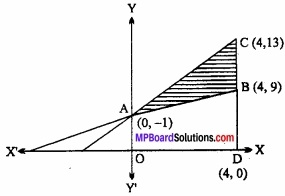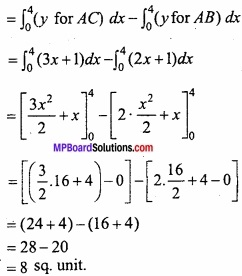# MP Board Class 12th Maths Important Questions Chapter 8 Application of Integrals

## MP Board Class 12th Maths Important Questions Chapter Application of Integrals

### Application of Integrals Important Questions

Application of Integrals Objective Type Questions

Question 1.

Question 1.
The value of $$\int_{0}^{\frac{\pi}{2}} \frac{\sin x}{\sin x+\cos x} d x$$ is:
(a) π
(b) $$\frac { \pi }{ 2 }$$
(c) $$\frac { \pi }{ 4 }$$
(d) $$\frac { -\pi }{ 4 }$$
(c) $$\frac { \pi }{ 4 }$$

Question 2.
Area of the best quadrant of the ellipse $$\frac { x^{ 2 } }{ a^{ 2 } }$$ + $$\frac { y^{ 2 } }{ b^{ 2 } }$$ = 1 is:
(a) πab
(b) $$\frac{1}{2}$$ πab
(c) $$\frac{1}{4}$$ πab
(d) $$\frac{1}{8}$$ πab
(c) $$\frac{1}{4}$$ πabQuestion 3.
The value of $$\int_{2}^{4} x^{3} d x$$ is:
(a) 60
(b) 50
(c) 70
(d) 256
(a) 60

Question 4.
$$\int_{0}^{2 a} f(x) d x=0$$, if:
(a) f(2a – x) = f(x)
(b) f(2a – x) = – f(x)
(c) f(x) is an even function
(d) f(x) is an odd function
(b) f(2a – x) = – f(x)

Question 5.
The value of $$\int_{0}^{2 \pi}|\sin x| d x$$ is equal to:
(a) 2
(b) $$\sqrt{3}$$
(c) 4
(d) 0
(d) 0

Question 2.
Fill in the blanks: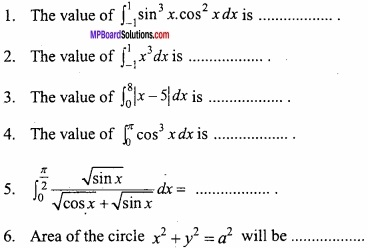1. 0
2. 0
3. 17
4. 0
5. $$\frac { \pi }{ 4 }$$
6. πa2

Question 3.
Write True/False: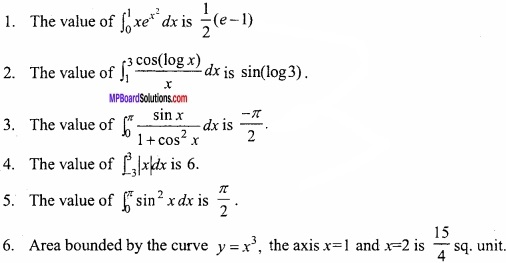1. True
2. True
3. False
4. False
5. True
6. True

Question 4.
Match the column:1. (b)
2. (a)
3. (d)
4. (c)
5. (f)
6. (e)

Question 5.
Write the answer in one word/sentence:1. 1
2. $$\frac { -\pi }{ 2 }$$ log 2
3. 2
4. $$\frac { \pi }{ 2 }$$
5. -4
6. $$\frac { \pi }{ 12 }$$

Application of Integrals Long Answer Type Questions – II

Question 1.
Find the area bounded by the parabolas x2 = 8y and y2 = 8x?
Solution:
Equation of given parabolas:
x2 = 8y ………………. (1)
and y2 = 8x ………………….. (2)
Solving eqns. (1) and (2),Coordinate of A(8, 8)
Two parabolas intersect at point O and A.
∴ Required area = Area OBALO – Area OCALOQuestion 2.
Find the area bounded by the curve y = cos x, the X – axis and x = 0, x = 2π, by integration method?
Solution:
y = f(x) = cos x
When x ∈ [0, $$\frac { \pi }{ 2 }$$, cos x ≥ 0;
x ∈ [0, $$\frac { \pi }{ 2 }$$, [0, $$\frac { 3\pi }{ 2 }$$, cos x ≤ 0;
When x ∈ $$\frac { 3\pi }{ 2 }$$, 2π] cos x ≥ 0Question 3.
(A) Find the area between the curves y2 = 4x and y = 2x? (Integral method)
Solution:
Given, Curve and line are y2 = 4x and – y = 2x
(2x)2 = 4x
⇒ 4x2 = 4x
⇒ x2 – x = 0
⇒ x (x – 1) = 0
∴ x = 0 or x = 1
Then y = 2x = 0 or y = 2 × 1 = 2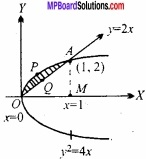The point of intersections of curve and line are (0, 0) and (1,2).
Area of shaded region = ar (OPAMO) – ar (OQAMO)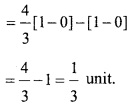(B) Find the area bounded by the curves x2 = 4y and x = 4y – 2 by integration method?
Solution:
Given, Curve and line are y2 = 4x and – y = 2x
(2x)2 = 4x
⇒ 4x2 = 4x
⇒ x2 – x = 0
⇒ x (x – 1) = 0
∴ x = 0 or x = 1
Then y = 2x = 0 or y = 2 × 1 = 2The point of intersections of curve and line are (0, 0) and (1,2).
Area of shaded region = ar (OPAMO) – ar (OQAMO)Question 4.
Find the area bounded by lines |x|+|y|= a by using integration method?
Solution:
The given lines are represented by |x|+|y| = a will be
x + y = a ……………. (1)
-x – y = a ……………………. (2)
x – y = a ……………………. (3)
and – x + y = a ………………… (4)
⇒ $$\frac { x }{ a }$$ + $$\frac { y }{ a }$$ = 1, $$\frac { x }{ -a }$$ + $$\frac { y }{ -a }$$ = 1
⇒ $$\frac { x }{ a }$$ + $$\frac { y }{ -a }$$ = 1, $$\frac { x }{ -a }$$ + $$\frac { y }{ a }$$ = 1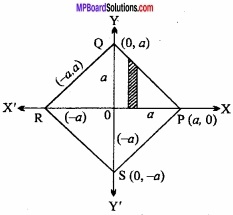The above graph is represented by the PQ, RS, PS and QR respectively.
Hence, the area bounded by these lines
= 4 × ∆Area of OPQ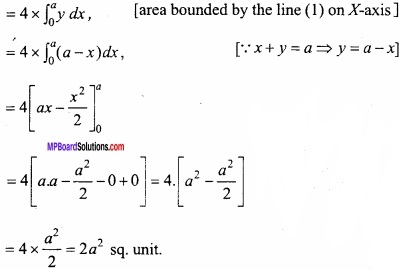Question 5.
(A) Find the rea of circle x2 + y2 = a2 by using integration method?
Solution:
Given:
x2 + y2 = a2
⇒ y2 = a2 – x2
⇒ y = $$\sqrt { a^{ 2 }-x^{ 2 } }$$
The circle is symmetric about the axes
Area = 4 × area of quadrant OAB(B)
Find the area of the circle x2 + y2 = 25 by using integration method?
Solution:
Solve as Q. No. 5 (A) by putting a = 5.

Question 6.
Find the area included between two parabolas:
y2 = 4 ax and x2 = 4ay, a > 0.
Solution:
The equations of the given parabolas are
y2 = 4ax.
and x2 = 4ay
Putting the value of y from eqn. (2) in eqn. (1), we haveFrom eqn.(2), we have
y = 0, y = $$\frac { (4a)^{ 2 } }{ 4a }$$ = 4a
∴The points of intersection of the given parabolas are (0, 0) and (4a, 4a).
Now, Required area = Area OBAL – Area OCALQuestion 7.
Find the area enclosed between parabolas y2 = 4x and x2 = 4y?
Solution:
y2 = 4x ……………… (1)
x2 = 4y …………………. (2)
Putting the value of y in eqn.(1), we get∴ At O, x = 0 and at M, x = 4
Hence Required area Area OAPB O
= Area OAPMO – Area OBPMOQuestion 8.
Find the area between curve y2 = x and x2 = y by integral method?
Solution:
Solve as Q.No. 7.

Question 9.
Find the area enclosed by the ellipse $$\frac { x^{ 2 } }{ a^{ 2 } }$$ + $$\frac { y^{ 2 } }{ b^{ 2 } }$$ = 1?
Solution:
Equation of the ellipse is∴ Required area = 4 area AOB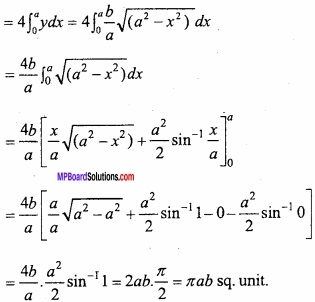Question 10.
Find the area of the region bounded by the parabola y2 = 4ax and its latus rectum?
Solution:
Equation of the parabola is
y2 = 4ax or y = ±2 $$\sqrt { ax }$$
In the figure, LSL’ is latus rectum and vertex is O (0, 0)
∴ Required area = 2 × Area OSLQuestion 11.
Find the area enclosed between parabola y2 = 4ax and straight line y = mx?
Solution:
Equation of the parabola y2 = 4ax ……………. (1)
Equation of straight line y = mx ………………….. (2)
O is the origin (O, O). P is the point of intersection of parabola and the straight line. Solving eqns. (1) and (2) we get,
y2 = 4ax
⇒ (mx)2 = 4ax
⇒ m2x2 – 4ax = 0
⇒ x(m2 – 4a) = 0
∴ x = 0, x = $$\frac { 4a }{ m^{ 2 } }$$Question 12.
Find the area bounded by the curve x2 = 4y, y = 2, y = 4 and Y – axis in the first quadrant? (NCERT)
Solution:
Given equation of curve is:
x2 = 4y
⇒ x = $$2\sqrt { y }$$
Required area = Area of ABCDQuestion 13.
Find the area of the smaller part of the circle x2 + y2 = a2 cut off by the line x = $$\frac { a }{ \sqrt { 2 } }$$? (NCERT)
Solution:
Given equation of curve is:
x2 + y2 = a2 …………………………. (1)
⇒ y2 = a2 – x2
⇒ y = $$\sqrt { a^{ 2 }-x^{ 2 } }$$
Equation of line is: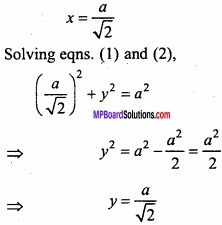Point of intersection of circle and line is A $$\frac { a }{ \sqrt { 2 } }$$, $$\frac { a }{ \sqrt { 2 } }$$
Coordinates of D is (a, 0).
∴ Required area = Area of ABD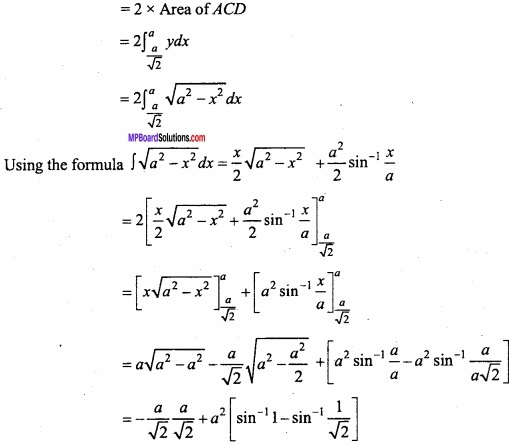Question 14.
The area between x = y2 and x = 4 is divided into two equal parts by the line x = a. Find the value of a? (NCERT)
Solution:
Given:
Equation of parabola is
x = y2
⇒ y = $$\sqrt{x}$$
Area bounded by the parabola and the line x = 4 is divided into two equal parts by the line x = a.
Area OEC = Area EFCBQuestion 15.
Find the area lying in the first quadrant and bounded by the circle x2 + y2 = 4 and the lines x = 0 and x = 2? (NCERT)
Solution:
Given equation of circle is:
x2 + y2 = 4
⇒ y2 = 4 – x2
⇒ y = $$\sqrt { (2)^{ 2 }-x^{ 2 } }$$Question 16.
Find the area enclosed by a circle x2 + y2 = 32, a line y = x and axis of A in the first quadrant?
Solution:
Equation of circle is:Putting the value of x in eqn. (2)
y = 4
The point of intersection of the line and circle are 0(0,0) and A(4,4) coordinates of B are are (4,0) and coordinates of C are (4$$\sqrt{2}$$,0).
Required area = Area OACBO
= Area OAB + Area ABC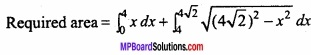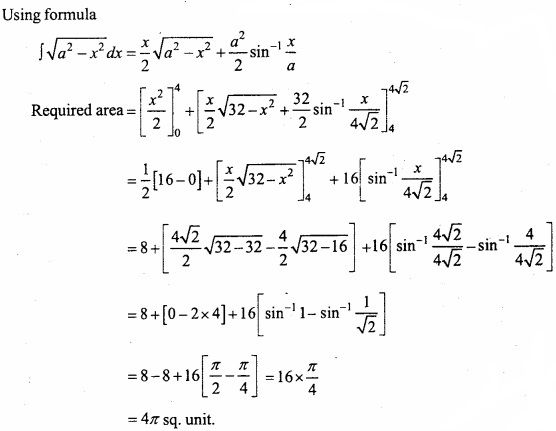Question 17.
Find the area of triangle whose sides are y = 2x + 1, y = 3x +1 and x = 4? (NCERT)
Solution:
Let the equation of sides AB,AC and BC of & ABC ore respectively.
y = 2x + 1 …………… (1)
y = 3x + 1 ………….. (2)
and x = 4 …………….. (3)
By solving eqns. (1) and (2) we have A (0, 1), by solving eqns. (1) and (3) we have B (4, 9) and by solving eqns. (2) and (3) we have C (4, 13).
Thus required area